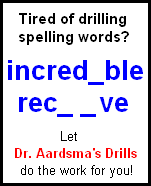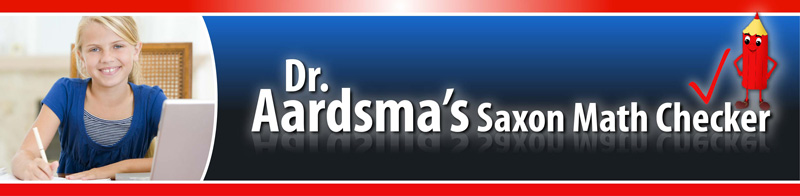Home
Convert 30-Day Trial to Full Registration
Change My Student's Lesson Number
Change the Question Skipping Behavior of My Student's Checker
Choose Problems to be Included in My Students' Lesson
Reset My Student's Current LessonTips for New Users
Use of Calculators in Saxon MathHow to Prepare for ACT/SAT
The Creator of Saxon Math## Tips For New Users

#### Tip 1: The Checker follows the answer which Saxon gives in the Saxon answer book very closely.

In fact, almost always, the Checker follows the Saxon answer exactly. In a VERY few cases the Checker deviates from the Saxon answer because, for example, the Saxon answer is wrong. In such cases, the Checker will usually inform you in the HINT field on the Checker's answer page (where students enter their answers) that the answer does not conform exactly to the Saxon answer.

So, when first learning to use the Checker, if you can't get the Checker to mark your answer right, first check with your teacher to see whether your answer is exactly the same as the Saxon answer. The Checker is looking for your answer to match the Saxon answer exactly.

#### Tip 2: The Checker is generally not fussy about spaces (but watch out for commas).

For example, if the correct answer is B + C = D, the Checker will accept B+C=D, or B + C=D, or B+C =      D, etc.

The only time a space must be present is when it would normally follow a comma. This is necessary for the Checker to be able to tell that you meant, for example, the two numbers 1, 234 and not the single number 1,234. The simple rule is: if the comma isn't part of a number, put a space after it.

#### Tip 3: The Checker's "helper buttons" (on the answer page) can be ignored until you get stuck trying to enter your answer.

If the answer you need to type in is 34, then ignore all the helper buttons on the answer page and just type 34 into the answer field. Click on the Submit button and you're on to the next problem.

But suppose the answer you need to type in is A × B = C. You can type the A, the B, the =, and the C. All of these characters are on your keyboard. But you can't type the × because your keyboard does not have a times sign. It has an x, but that is the letter x, for spelling ox and fox, not a times sign. The Checker furnishes you with buttons on its answer page for special characters like the times sign. These software buttons act just like more keys on your keyboard.

Your keyboard has other limitations. For example, in math, Y squared is typed using a superscript 2. Your keyboard lacks ability to type superscripts. Or the answer may involve a subscript---for example: N subscript W = 5. Or the answer may be a fraction. Your keyboard lacks ability to type fractions.

In all such cases, the Checker furnishes "helper buttons" so you can enter your answer using your limited keyboard. For the N subscript W = 5 case, using the helper button for subscript W will give this result in the answer field: N{_W} = 5. That's its way of telling the Checker that the W is a subscript.

#### Summary of the Basics

To make your answers recognizable by the Checker, use this procedure. First, simply try to type the answer just the way it appears in your own solution. If you get stuck (for example, because the answer has a Y squared in it, and superscript 2 is not a key on the keyboard) use the Checker helper buttons to get unstuck. That's the only time you need use the helper buttons---to get unstuck.

#### Tip 4: If you get the answer wrong the first time, you can use your browser's back button to go back to your answer and change it.

[This tip does not work with all browsers. It works with Internet Explorer and Microsoft Edge, but it does not work, for example, with Chrome or Firefox.]

Answers can get lengthy, especially in the higher grades. It can be frustrating to have to type a lengthy answer over again because you made a typing error the first time and the Checker marked it wrong. So don't type it over. Use the back button to go back to your answer and change it.

This is also a great time saver when a question has more than one possible right answer and you're not sure which answer the Checker wants.

That is, sometimes an answer can be written in more than one mathematically correct way. For example, if 1 + 2 = 3 is the Saxon answer to the problem, then 2 + 1 = 3 is probably also a mathematically correct answer. For some problems, the answer can be written correctly in many different ways.

The Checker only accepts the Saxon answer book answer. If the Saxon answer book has 1 + 2 = 3, then you have to enter your answer as 1 + 2 = 3; the Checker will mark 2 + 1 = 3 wrong, even though it is mathematically right.

This is not much of a problem in practice. The Checker has a HINT field on the answer page. In the case of answers which can be written in many different ways, the Checker will give you a hint so you will know the correct way to choose.

Sometimes the answer can be written in two ways and there will be no hint. In most instances, you will know from experience what choice you should make. But if you are unsure, try it one way. If you get it wrong, use the back button on your browser and change your answer to the other way.

#### Tip 5: The button that says "E-mail teacher to check this one" is for you to use whenever the Checker won't accept your answer and you don't know what else to do.

You should use this button to move on after a couple of failed attempts, rather than sitting there frustrated and stuck. You may be missing something very trivial (like that the abbreviation for inches ends with a period, which you have left out), or it is even possible that the Checker has the wrong answer, due to a typing error in its database. It is simply not worth wasting time or getting frustrated in such instances. Use the "E-mail teacher to check this one" button so you and your teacher can work together later to quickly figure out what the problem was.